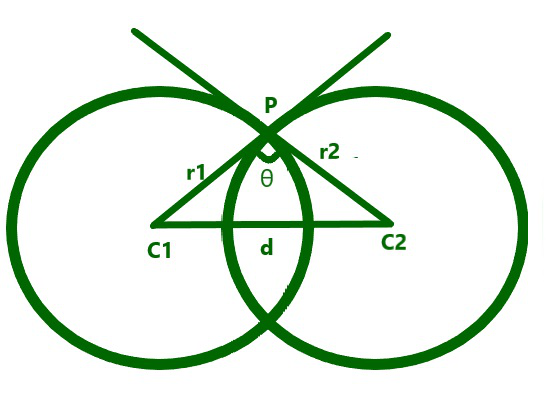Open In App

# Angle of intersection of two circles having their centers D distance apart

Given two positive integers R1 and R2 representing the radius of two intersecting circles having a distance D between their centers, the task is to find the cosine of the angle of intersection between the two circles.

Examples:

Input: R1 = 3, R2 = 4, D = 5
Output: 0

Input: R1 = 7, R2 = 3, D = 6
Output: 0.52381

Approach: The given problem can be solved by using the Geometric Algorithm as illustrated below:From the above image and using the Pythagoras Theorem, the cosine of the angle of intersection of the two circles can be found using the formula:Below is the implementation of the above approach:

## C++

 // C++ program for the above approach#include using namespace std; // Function to find the cosine of the// angle of the intersection of two// circles with radius R1 and R2float angle(float R1, float R2, float D){    float ans = (R1 * R1 + R2 * R2 - D * D)                / (2 * R1 * R2);     // Return the cosine of the angle    return ans;} // Driver Codeint main(){    float R1 = 3, R2 = 4;    float D = 5;    cout << angle(R1, R2, D);     return 0;}

## Java

 // Java program for the above approachimport java.io.*; class GFG{     // Function to find the cosine of the// angle of the intersection of two// circles with radius R1 and R2static float angle(float R1, float R2, float D){    float ans = (R1 * R1 + R2 * R2 - D * D) /               (2 * R1 * R2);                    // Return the cosine of the angle    return ans;} // Driver Codepublic static void main (String[] args){    float R1 = 3, R2 = 4;    float D = 5;         System.out.println(angle(R1, R2, D));}} // This code is contributed by Ankita saini

## Python3

 # Python3 program for the above approach # Function to find the cosine of the# angle of the intersection of two# circles with radius R1 and R2def angle(R1, R2, D):         ans = ((R1 * R1 + R2 * R2 - D * D) /            (2 * R1 * R2))     # Return the cosine of the angle    return ans # Driver Codeif __name__ == '__main__':         R1 = 3    R2 = 4    D = 5         print(angle(R1, R2, D))     # This code is contributed by ipg2016107

## C#

 // C# program for the above approachusing System; class GFG{     // Function to find the cosine of the// angle of the intersection of two// circles with radius R1 and R2static float angle(float R1, float R2, float D){    float ans = (R1 * R1 + R2 * R2 - D * D) /               (2 * R1 * R2);                    // Return the cosine of the angle    return ans;} // Driver Codepublic static void Main(string[] args){    float R1 = 3, R2 = 4;    float D = 5;         Console.Write(angle(R1, R2, D));}} // This code is contributed by rutvik_56.

## Javascript

 

Output:

0

Time Complexity: O(1)
Auxiliary Space: O(1)